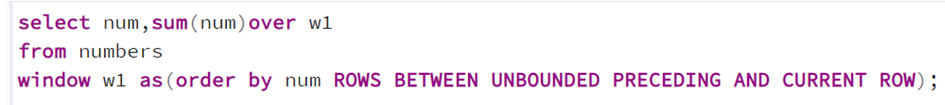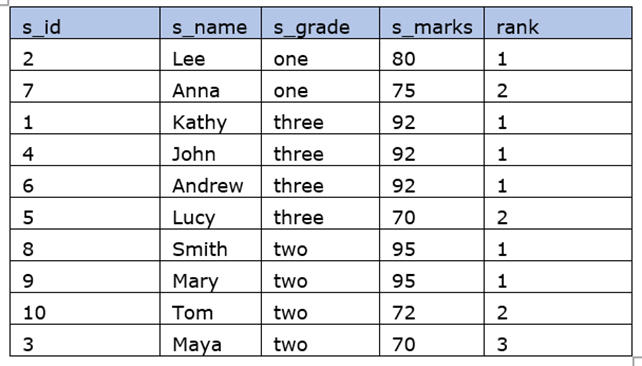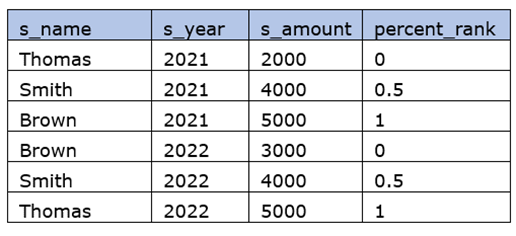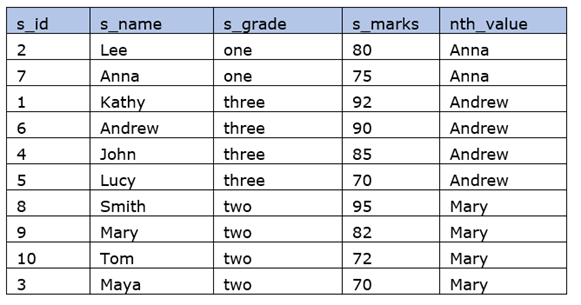top of pageSearch

# PostgreSQL Window functions

Window functions compute operations on a set of related rows called window frame, in a dataset. This is related to the calculations done by aggregate functions in SQL. But window functions are more powerful in such a way that,

Unlike regular aggregate functions in SQL, the window function does calculations for each row in the window and the individual rows in the window are accessible.

Normal aggregate calls would cause the grouping of rows into a single output row and individual record-level data will not be accessible for further use.

The difference in working with aggregate functions and window functions with an example.

We have a table student with records of 10 students of three different grades one, two, and three.If we want to get the average marks of students from each grade and the number of students in each grade, we should use the query,Result:When we use GROUP BY clause, the individual records of each grade are flattened into a single row, and we get average marks and the number of students in each grade. We will not get other individual records like a student's name or the number of students in a particular grade etc.

Let us use the window function to execute the following statement,Output is,over(partition by s_grade) clause defines the groups of records that are of the same grade. The window functions avg() and count() creates aggregates in the corresponding group of records without flattening individual records into a single row. Window functions give records of each row in the window.

Logical order of operations in SQL

The order in which SQL queries are processed is given below.

1. FROM, JOIN

2. WHERE

3. GROUP BY

4. Aggregate functions

5. HAVING

6. Window functions

7. SELECT

8. DISTINCT

9. UNION/INTERSECT/EXCEPT

10. ORDER BY

11. OFFSET

12. LIMIT/FETCH/TOP

Please note that we cannot use window functions anywhere in the WHERE, GROUP BY clause, etc., since the window functions are processed only after them. Window functions are allowed only in the SELECT and the ORDER BY clause in a query.

Window functions syntax

Window functions are defined by the OVER clause. Three main things we should know about before defining window functions are,

1. What records will be in the window frames so that the OVER clause can compute them across the window frame

2. How the records are ordered.

3. The upper and lower bounds of the window frame.

Syntax:

window_function_name (expression) OVER (

PARTITION_clause

ORDER_clause

FRAME_clause

)

window_function_name:

Name of the window function such as avg(), max(), rank(), etc.

OVER clause:

Windows are defined by the OVER clause and it directly follows the window function name. OVER clause distinguishes between non-window aggregate functions and window functions.

Partition_clause:

PARTITION BY is a subclause of the OVER clause that subdivides rows into groups called partitions, to which the window function is applied. The records in each group share values that the expression in the PARTITION BY clause tells. If there is no PARTITION BY clause, then the entire records will be considered as a partition.

Syntax: PARTITION BY expression1, expression2, ...

We are going to find the average marks of students in each grade using the window function avg().Output is,Here, we can see that all rows in the table have individual output rows. The average of marks is taken across a group of records that shares the same grade value.

While processing each row, say it as the current row, window frames are generated based on the PARTITION BY clause. Since we have 3 grade-values are there in the table, 3 different window frames are generated. Different window frames are highlighted below.ORDER clause:

ORDER BY within the OVER clause can control the order in which each record is processed.

Syntax: ORDER BY

expression [ASC | DESC] [NULL {FIRST| LAST}]

,...

Let us see an example,

We are going to find the rank of students in each grade based on marks.Result:We don’t need to pass a parameter for the rank() function, it generates a numerical rank for each record in the window frame of the current row when it is processed. Using different OVER clauses, we can use multiple window functions in a query that divides the records in different ways.

The frame_clause

This optional clause defines window frame bounds with rows. Frame clause allows us to manage partitions differently.

ROWS and RANGE subclauses in the OVER clause specify the window frame bounds. We use the frame clause only if the ORDER BY clause is also present.

The frame clause has two forms:

ROWS BETWEEN start_point AND end_point

ROWS BETWEEN start_point AND end_point

Syntax:

{ RANGE | ROWS } frame_start

{ RANGE | ROWS } BETWEEN frame_start AND frame_end.frame_start options are, N PRECEDING, UNBOUNDED PRECEDING, or CURRENT ROW

frame_end options are, CURRENT ROW, UNBOUNDED FOLLOWING, or M FOLLOWING

We must make sure the frame start is less than the frame end.

ROWS BETWEEN start_point AND end_point

Let us look at an example to get a better idea of it.

We have a table,

Table: NumbersWe run the query using the named window w1, using the WINDOW clause,Since we give the frame start option as UNBOUNDED PRECEDING and the frame end option as CURRENT ROW, the sum calculation happens like this. ORDER BY clause will order the data in the window.The UNBOUNDED PROCEDING pointer is always at the first record since we have given it as the frame start option.

During the processing of records, when the first row is the current row, it gives a sum as 1 since the window frame start and current row both are at the same row. When the second row becomes the current row, now the window frame starts in the first row and the sum is calculated with numbers in the new window frame.

Now we will see another example with the window frame start option as N PRECEDING, where we give a value for N that says how many records should precede the current row in the window frame.

When we run the query,Output is,Here window start option we have given is 2 PRECEDING, window range will start from 2 records preceding the current row.

The sum calculation is like this,RANGE BETWEEN start_point and end_point

Here we consider a range of values while processing the current row. Let us check this with an example.

We have a table number_dup which has duplicate values it.We will run the queries with the only difference of subclauses ROWS and RANGE and check it out.

Query with ROWS subclause:The sum is calculated as current num+ previous num (1 record preceding the current row). Each record will be processed individually.

Query with RANGE subclause:Here RANGE combines all the rows it comes across with values including duplicates, rather than processing them one at a time. PostgreSQL splits the window function into frames using the ORDER BY clause and then aggregates the data among those frames.

In the above example, let us take the current row as 4,The sum of row number 4 is calculated as the sum of row number 1 + row number 2 + row number 3 + row number 4.

List of Window functions

Below is the list of Window functions used in PostgreSQL.

Aggregate Functions

avg()

count()

max()

min()

sum()

Ranking Functions

row_number()

rank()

dense_rank()

Distribution Functions

percent_rank()

cume_dist()

Analytic Functions

lag()

ntile()

first_value()

last_value()

nth_value()

Let us have a look at the window functions with examples.

avg(expression)

This window function computes the average value for rows within the window frame.

Here we are finding the average student's marks in each grade,output is,count(expression)

count() calculates the count of values for rows within the window frame.

We will find the count of students in each grade in the student table.Output is,max(expression)

max() calculates the maximum value within the window frame.

Let us find the student’s name and marks who has got the maximum marks in each grade,output is,min(expression)

min() calculates the minimum value within the window frame.

Here we are going to find the minimum marks in each grade using the named window function.output is,sum(expression)

max() calculates the sum of values within the window frame.

Let us do this example with another table.

Table: Student_marksWe are finding the total marks of each student from term and term2.Result is,row_number()

row_number() assigns a sequential integer number for each row within the partition.

The row number starts with 1. PARTITION BY clause is optional and since the row_number() function is order sensitive, the ORDER BY clause is mandatory.

Let us use this function to get row numbers for the table student_marks.Result is,rank()

rank() returns the rank of values in a partition based on the ORDER BY expression in the OVER clause.

Let us find the rank of students based on the marks in each grade using the named window function.Result is,dense_rank()

dense_rank() returns a ranking within the partition, with no gaps and the same ranking for tied values.

To know this better, we have updated the table student with tied values. Highlighted are the tied values for marks in each grade.When we use the rank() function in this table,Result is,Since there are tied values, we are not getting the rank values in sequential order. For grade three, the rank comes as 1,1,1,4. Rank 2,3 are missing.

For grade two, rank comes as 1,1, and 3. Rank 2 is missing.

Let us see how it works with the dense_rank() function.Here there is no gap between ranks even though there are tied values. Dense_rank() generates a contiguous sequence of ranks.

When we run the query, the result ispercent_rank()

the percentile ranking number of a row—a value in [0, 1] interval:

(Rank - 1) / (number of rows in window partition- 1). The result would be between 0 and 1.

Table: salesWe are finding the percentile ranking based on year.cume_dist()

The cumulative distribution of a value within a group of values, i.e., the number of rows with values less than or equal to the current row’s value divided by the total number of rows. A value in (0, 1] interval is the result.The lead() function provides access to the following row. We can provide the offset (number of rows forward from the current row )and default value also.

Offset and default are optional.

Let us check this example,Output is,This returns NULL when there is no following row within the partition.

Let us check another example that passes offset and default values.Output is,lag(expression, offset, default)

lag() function provides access to the previous row. We can provide the offset number that specifies the position of the row that comes before the current row. Default value also can be passed.

An example is given below.This returns NULL values when there is no record found within the partition. Here the records are checked within the year group.When we pass offset,ntile(n)

ntile() function divides rows within an ordered partition into n number of roughly equal groups, and assigns each row its group number.Here ntile() function divides the records into 3 groups of 2,2, and 1 rows.Since we haven’t given PARTITION BY clause, all records will be considered as a partition and divided into n number of groups.

first_value(expression)

This function returns the value for the first row within an ordered partition.

For example,Here, the first()value function fetches the name of the student who has high marks in each department by returning the first name from the records which are sorted in descending order. The expression we pass to this function is the column name we want.

last_value(expression)

This function returns the value for the last row within an ordered partition.

For example,Result is,Here, the last()value function fetches the name of the student who has got fewer marks in each department by returning the last name from the records which are sorted in descending order.

We use RANGE BETWEEN UNBOUNDED PRECEDING AND UNBOUNDED FOLLOWING that defines the frame starting from the first row of the partition and the frame ending at the last row of the partition.

With the default window frame for ORDER BY, RANGE UNBOUNDED PRECEDING, last_value() returns the value for the current row.

nth_value(expr, n)

It returns the value for the nth row within the window frame. The value of n should be an integer.

Let us find out the name of the student who has got the second highest marks in each grade with the example below.And that was an overview of all window functions used in PostgreSQL. Thank you for reading.

Happy learning!!!

162 views1 comment

## Recent Posts

See All

Snowflake is a cloud native database and can be hosted on any of the clouds namely AWS, GCP or Azure. While using snowflake we pay for only the storage we use and the compute which was actually used d

bottom of page